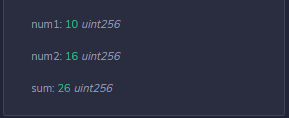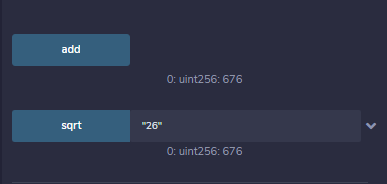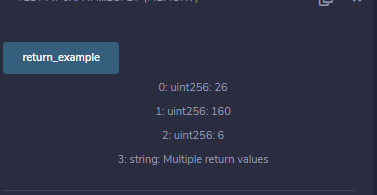# Solidity – Functions

• Difficulty Level : Basic
• Last Updated : 11 May, 2022

A function is basically a group of code that can be reused anywhere in the program, which generally saves the excessive use of memory and decreases the runtime of the program. Creating a function reduces the need of writing the same code over and over again. With the help of functions, a program can be divided into many small pieces of code for better understanding and managing.

### Declaring a Function

In Solidity a function is generally defined by using the function keyword, followed by the name of the function which is unique and does not match with any of the reserved keywords. A function can also have a list of parameters containing the name and data type of the parameter. The return value of a function is optional but in solidity, the return type of the function is defined at the time of declaration.

```function function_name(parameter_list) scope returns(return_type) {
// block of code
}
```

 `// Solidity program to demonstrate ``// function declaration``pragma solidity ^0.5.0;`` ` `// Creating a contract``contract Test {`` ` `   ``// Defining function to calculate sum of 2 numbers``   ``function` `add() public view returns(uint){``      ``uint num1 = 10; ``      ``uint num2 = 16;``      ``uint sum = num1 + num2;``      ``return` `sum;``   ``}``}`

Output :In the above example, you must have seen the usage of “view”. View in a function ensures that they will not modify the state of the function. The following statements if present in the function will modify the state of the function and the compiler will throw a warning.

• Modifying state variables.
• Emitting events.
• Creating other contracts.
• Using self-destruct.
• Sending Ether via calls.
• Calling any function which is not marked pure or view.
• Using inline assembly containing certain opcodes.
• Using low-level calls.

### Function Calling

A function is called when the user wants to execute that function. In Solidity the function is simply invoked by writing the name of the function where it has to be called. Different parameters can be passed to function while calling, multiple parameters can be passed to a function by separating with a comma.

 `// Solidity program to demonstrate``// function calling``pragma solidity ^0.5.0;`` ` `// Creating a contract``contract Test {`` ` `   ``// Defining a public view function to demonstrate``   ``// calling of sqrt function``   ``function` `add() public view returns(uint){``      ``uint num1 = 10; ``      ``uint num2 = 16;``      ``uint sum = num1 + num2;``      ``return` `sqrt(sum); ``// calling function``   ``}`` ` `   ``//Defining public view sqrt function``   ``function` `sqrt(uint num) public view returns(uint){``       ``num = num ** 2;``       ``return` `num;``   ``}``}`

Output :### Return Statements

A return statement is an optional statement provided by solidity. It is the last statement of the function, used to return the values from the functions. In Solidity, more than one value can be returned from a function. To return values from the function, the data types of the return values should be defined at function declaration.

 `// Solidity program to demonstrate ``// return statements``pragma solidity ^0.5.0;`` ` `// Creating a contract``contract Test {`` ` `   ``// Defining a public view function to``   ``// demonstrate return statement``   ``function` `return_example() public view returns(uint, uint, uint, string memory){``      ``uint num1 = 10; ``      ``uint num2 = 16;``      ``uint sum = num1 + num2;``      ``uint prod = num1 * num2;``      ``uint diff = num2 - num1;``      ``string memory msg = ``"Multiple return values"``;``      ``return` `(sum, prod, diff, msg); ``   ``}``}`

Output :My Personal Notes arrow_drop_up